# Quantum Computing

Quantum computing is attracting a lot of attention recently due to significant advances in constructing quantum devices. While this does not yet mean that large scale quantum computing is possible, it might be so in the future. Thus, for the time being, quantum simulators play an important role for inventing and testing of algorithms.

Quantum computing requires a different approach to programming than many of us are used to on classical computers. The more or less standard approach is now via so-called quantum circuits, a generalisation of classical logical circuits (or better classical reversible circuits). The basic entity of such a circuit is a quantum state composed out of qubits. Such states can be manipulated via unitary quantum gates. Classical information can be retrieved by performing (projective) measurements.

For a comprehensive introduction and discussion see (Nielsen and Chuang 2016).

# qsimulatR Package

By the nature of quantum mechanics, quantum algorithms are probabilistic algorithms. As such, R could be the natural language to analyse the corresponding results. The package qsimulatR presented here provides a quantum simulator which allows one to implement quantum circuits in R. qsimulatR uses S4 classes to implement quantum state manipulations intuitively via multiplications *.

General single qubit gates and general controlled single qubit gates can be easily defined. For convenience, it currently directly provides the most common gates (X, Y, Z, H, Z, S, T, Rx, Ry, Rz, CNOT, SWAP, toffoli or CCNOT, CSWAP). qsimulatR supports plotting of quantum circuits.

Since one important platform for real quantum devices is given by qiskit, qsimulatR is able to export circuits into qiskit. The such exported code can in principle be run directly on IBM’s real quantum hardware.

We have developed qsimulatR for a lecture on Quantum Computing taught at the University of Bonn, Germany, during winter term 2020. It is available on CRAN since last week. The most up-to-date version can be obtained from the github repository.

## A quantum state

The basis for the simulator is a quantum state, called qstate in qsimulatR realised as an S4 class. One can generate a qstate with a number of qubits (here 2 by nbits=2) as follows

library(qsimulatR)
x <- qstate(nbits=2)
x
   ( 1 )    * |00> 

Per default this state is in the computational basis with all qubits in the $$|0\rangle$$ state. The convention is that the least significant bit is printed as the rightmost qubit, the most significant one as the leftmost qubit. In front of the basis state the complex amplitude of this state is printed.

The basis state can also be supplied to qstate as an optional argument, e.g.

CatInBox <- qstate(nbits=1, basis=c("|dead>", "|alive>"))
CatInBox
   ( 1 )    * |dead> 

and also the coefficients of the separate basis states can be set, even though that is not realistic on a real quantum device and should be avoided for other than testing purposes

CatInBox <- qstate(nbits=1, basis=c("|dead>", "|alive>"),
coefs=as.complex(c(1/sqrt(2), 1/sqrt(2))))
CatInBox
   ( 0.7071068 )    * |dead>
+ ( 0.7071068 )    * |alive> 

Quantum circuits can be visualised very easily. Each qubit is represented by a horizontal line. In qsimulatR a circuit can be plotted by plotting a qstate object. If no gate was applied yet, the corresponding image is not very interesting

plot(x, qubitnames=c("qubit1", "qubit2"))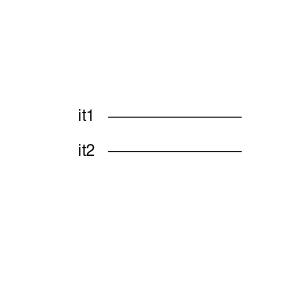## Applying single qubit gates

As said above, quantum states can be manipulated via unitary gates. For this the * operator is overloaded for certain types of gates applied to qstate objects. One important gate is the so-called Hadamard gate, which has the matrix representation $H\ =\ \frac{1}{\sqrt{2}}\begin{pmatrix} 1 & 1 \\ 1 & -1 \\ \end{pmatrix}\,.$ The Hadamard gate is available in qsimulatR as H(j). Every single qubit gate needs to be supplied with the information j to which qubit it is to be applied to. The application to the first qubit (i.e. the least significant one) looks as follows:

x <- qstate(nbits=2)
y <- H(1) * x
y
   ( 0.7071068 )    * |00>
+ ( 0.7071068 )    * |01> 

In R we start counting with 1, which corresponds to the least significant bit (or the rightmost one in $$|00\rangle$$). H(1) returns an S4 object of class sqgate and the multiplication with qstate is overloaded.

Now, with a gate included, plotting the state represents the quantum circuit used to generate the state, for instance

plot(y)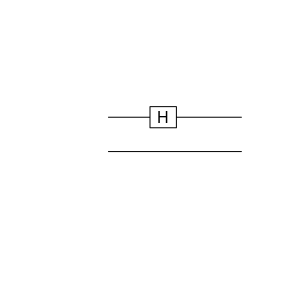Even if there is a list of predefined standard single qubit gates (H, X, Z, Y, Rz, Ry, Rz, Id, S, Tgate), any single qubit gate can be defined as follows via its matrix representation

myGate <- function(bit) {
methods::new("sqgate", bit=as.integer(bit),
M=array(as.complex(c(1,0,0,-1)), dim=c(2,2)),
type="myGate")
}

From now on this gate can be used like the predefined ones

z <- myGate(1) * y
z
   ( 0.7071068 )    * |00>
+ ( -0.7071068 )   * |01> 

The argument M must be a unitary $$2\times 2$$ complex valued matrix, which in this case is the third Pauli matrix predefined as Z. If M is not unitary, an error will the thrown.

### Truth Table

Sometimes it is useful to look at the quantum analogue of a truth table of a gate as it immediately shows how every qubit is influenced depending on the other qubits. It can provide a consistency check for a newly implemented gate, too. The truth table of a single qubit gate (here the X gate) can be obtained as follows

truth.table(X, 1)
  In1 Out1
1   0    1
2   1    0

## Multi-qubit gates

For universal quantum computing more than single qubit gates are necessary. The most important two-qubit gate is the controlled NOT or CNOT gate. It has the truth table

truth.table(CNOT, 2)
  In2 In1 Out2 Out1
1   0   0    0    0
2   0   1    1    1
3   1   0    1    0
4   1   1    0    1

and can for instance be used as follows to generate a Bell state

z <- CNOT(c(1,2)) * y
z
   ( 0.7071068 )    * |00>
+ ( 0.7071068 )    * |11> 

which is maximally entangled. Plotting the resulting circuit looks as follows

plot(z)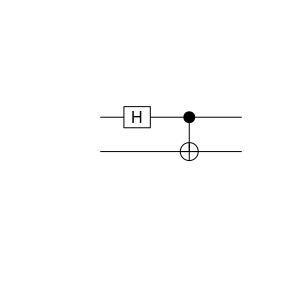The arguments to CNOT are the control and target bit in this order. Again, arbitrary controlled single qubit gates can be generated as follows

CX12 <- cqgate(bits=c(1L, 2L), gate=X(2L))
z <- CX12 * y
z
   ( 0.7071068 )    * |00>
+ ( 0.7071068 )    * |11> 

which in this case is the CNOT gate again with control bit 1 and target bit 2. cqgate is a convenience function to generate a new S4 object of S4 class cqgate. Note that a similar function sqgate for single qubit gates is available. The gate argument to cqgate can be any sqgate object.

Another widely used two-qubit gate is the SWAP gate, which swaps two qubits, in the following example the first and the second qubit:

z <- SWAP(c(1,2)) * y
z
   ( 0.7071068 )    * |00>
+ ( 0.7071068 )    * |10> 
plot(z)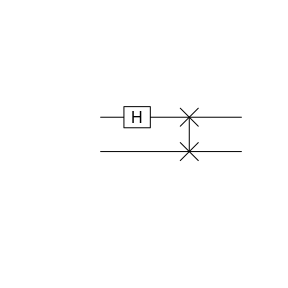The order of the bits supplied to SWAP is irrelevant, of course

z <- SWAP(c(2,1)) * y
z
   ( 0.7071068 )    * |00>
+ ( 0.7071068 )    * |10> 

Finally, there are also the controlled CNOT or Toffoli gate and the controlled SWAP or Fredkin gate available. The next code snipped shows the application of the CCNOT gate.

x <- H(1) *(H(2) * qstate(3))
x
   ( 0.5 )  * |000>
+ ( 0.5 )  * |001>
+ ( 0.5 )  * |010>
+ ( 0.5 )  * |011> 
y <- CCNOT(c(1,2,3)) * x
y
   ( 0.5 )  * |000>
+ ( 0.5 )  * |001>
+ ( 0.5 )  * |010>
+ ( 0.5 )  * |111> 
plot(y)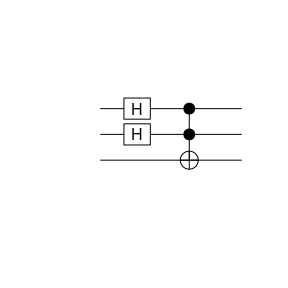The arguments to CCNOT is a vector of three integers. The first two integers are the control bits, the last one the target bit.

Similarly, the CSWAP or Fredkin gate

y <- CSWAP(c(1,2,3)) * x
y
   ( 0.5 )  * |000>
+ ( 0.5 )  * |001>
+ ( 0.5 )  * |010>
+ ( 0.5 )  * |101> 
plot(y)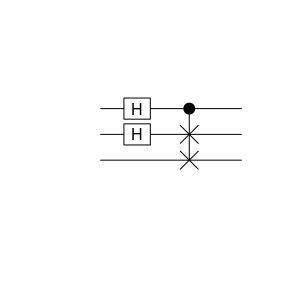## Multi-gate circuits as functions

You might want to use specific circuits of several gates more than once and therefore find it tedious to type it completely every time you use it. Defining a new gate combining all of the operations might not be as easy either. In these cases we find it convenient to define generating functions of the form

myswap <- function(bits){
function(x){
CNOT(bits) * (CNOT(rev(bits)) * (CNOT(bits) * x))
}
}

where an outer function takes any required information concerning the circuit. It then returns a function that applies given circuit to a state.

Our example above implements a SWAP using only CNOT gates as can be checked with the truth table:

truth.table(myswap, 2)
  In2 In1 Out2 Out1
1   0   0    0    0
2   0   1    1    0
3   1   0    0    1
4   1   1    1    1

We can plot the circuit in the way we are used to as well.

circuit <- myswap(1:2)
plot(circuit(qstate(2)))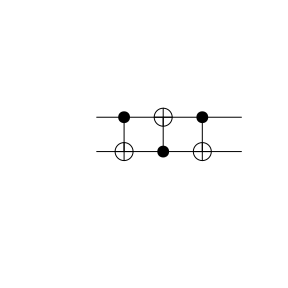## Measurements

At any point single qubits can be measured

res <- measure(y, 1)
summary(res)
Bit 1 has been measured 1 times with the outcome:
0:  1
1:  0 
plot(res$psi)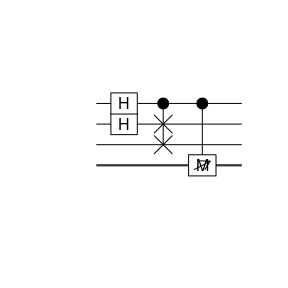In this case the third qubit is measured and its value is stored in res$value. The wave function of the quantum state collapses, the result of which is stored again as a qstate object in res$psi. Such a measurement can be repeated, say 1000 times, for qubit 3 rv <- measure(y, 3, rep=1000)$value
hist(rv, freq=FALSE, main="Probability for Qubit 3")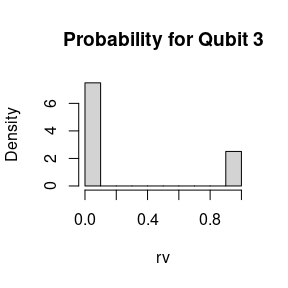where we read of from the wave function that the ratio should be $$3:1$$ for values $$0$$ and $$1$$, which is well reproduced.

## Quantum Fourier Trafo and other higher level functionality

The quantum Fourier transformation (qFT) is an important building block of many algorithms. qsimulatR provides an implementation of qFT over $$\mathbb{Z}_{2^n}$$ via the qft function.

y <- qstate(3)
y <- qft(y, inverse=FALSE)
plot(y)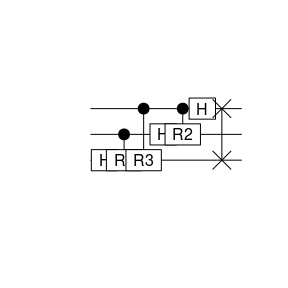qft can also apply the inverse qFT by setting inverse=TRUE. Moreover, the qFT can also be applied to a subset of qubits only, here to qubits two to four while the first qubit is left alone:

y <- qstate(4)
y <- qft(y, bits=c(2:4), inverse=FALSE)
plot(y)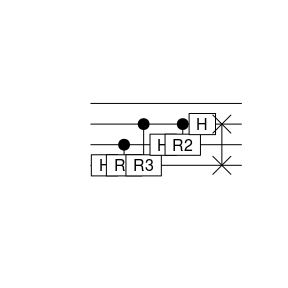For more details we refer to the qft vignette. Based on the qFT algorithm the phase estimation algorithm can be defined. It is implemented in qsimulatR as the function phase_estimation. The phase estimation allows to estimate the phase of eigenvalues of unitary operators (all eigenvalues $$\lambda=e^{2\pi i\varphi}$$ of unitary operators $$U$$ have modulus 1, i.e. $$|\lambda| = 1$$). In order to call the phase_estimation function, a wrapper function for the unitary operator needs to be defined, e.g. for the NOT operator, which is the Pauli X matrix $X\ =\ \begin{pmatrix} 0 & 1 \\ 1 & 0 \\ \end{pmatrix}$ The wrapper function then needs to implement a controlled NOT operation, i.e. the CNOT operator, to the power $$2^j$$. $$X^2=1$$, thus we can implement it as follows

cnotwrapper <- function(c, j, x, k) {
if(j == 1) return(CNOT(c(c, k)) * x)
return(Id(k) * x)
}

For the phase_estimation function, this wrapper function must have the control qubit c as first argument, as the second the integer power j and as the third the qstate object x. Additional parameters to the wrapper function can be passed via the ... argument to phase_estimation.

X has eigenvalues $$\lambda_\pm=\pm1$$. Thus, the corresponding phases are $$\varphi = 0$$ and $$\varphi= 1/2$$. Both can be represented as binary fractions with a single bit exactly. Here we use $$t=2$$ qubits in the call of phase_estimation determined by the length of the bitmask argument to the function. In addition, the phase estimation procedure needs a trial state. If possible, this trial state should be in an eigenstate of the unitary operator. However, also a random superposition of eigenstates works. In the example, we use the first qubit as the trial state, which we initialise in the state $$(|0\rangle + |1\rangle)/\sqrt{2}$$, which is an eigenstate of $$X$$ with eigenvalue $$+1$$. Qubits two and three are used in the phase estimation function

x <- H(1) * qstate(3)
x <- phase_estimation(bitmask=c(2:3), FUN=cnotwrapper, x=x, k=1)
x
   ( 0.7071068 )    * |000>
+ ( 0.7071068 )    * |001> 

How to interpret the result? The first qubit stays in the state $$(|0\rangle + |1\rangle)/\sqrt{2}$$, as it is an eigenstate. The other two qubits encode the phase $$\varphi=0.00$$ in binary fraction representation. Using the other eigenstate $$(|0\rangle - |1\rangle)/\sqrt{2}$$ leads to

x <- H(1) * (X(1) * qstate(3))
x <- phase_estimation(bitmask=c(2:3), FUN=cnotwrapper, x=x, k=1)
x
   ( 0.7071068 )    * |100>
+ ( -0.7071068 )   * |101> 

and $$\varphi=0.10 = 1/2$$. More details and a more complex example can be found in the qsimulatR vignette on phase_estimation.

# Quantum Noise

Current quantum hardware is noisy. qsimulatR offers possibilities to simulate such noise. First of all, there is a noisy gate with different noise models. With a certain probability p (default value 1) a gate is applied to qubit bit, which can follow the models X, Y, Z, small or any:

• X, Y or Z are the corresponding Pauli gates
• in case of small, an almost unit gate is applied with a small deviation parametrised by and additional parameter sigma representing the standard deviation of Gaussian noise
• any means an arbitrary, uniformly sampled, SU(2)-matrix is applied

Examples are

x <- noise(bit=1, error="X") * qstate(nbits=2)
x
   ( 1 )    * |01> 
y <- noise(bit=2, p=0.5) * x
y
   ( 0.5139376+0.0725443i ) * |01>
+ ( 0.8542451-0.0295094i ) * |11> 
z <- noise(bit=2, error="small", args=list(sigma=0.1)) * x
z
   ( 0.9848509+0.1627725i ) * |01>
+ ( -0.04433343-0.04010527i )  * |11> 

This is generalised to a quantum state in qsimulatR by making it a property of a state as follows:

x <- qstate(nbits=2, noise=genNoise(nbits=2, p=1, error="any"))

Now, a random gate is applied with every gate applied to such a quantum state, e.g.

y <- Id(2) * x
y
   ( 0.5140889+0.8017019i ) * |00>
+ ( -0.0753246+0.2954876i )    * |10> 

See the function genNoise for more details.

# Export to Qiskit

IBM provides quantum computing platforms, which can be freely used. However, one needs to programme in python using the qiskit package. For convenience, qsimulatR can export a circuit translated to qiskit

filename <- paste0(tempdir(), "/circuit.py")
export2qiskit(y, filename=filename, import=TRUE)
Warning in export2qiskit(y, filename = filename, import = TRUE): Gate of type
'Id' is not supported!
Warning in export2qiskit(y, filename = filename, import = TRUE): Gate of type
'ERR' is not supported!

which results in the following file

cat(readLines(filename), sep = '\n')
# automatically generated by qsimulatR
import numpy as np
from qiskit import(QuantumCircuit, execute, Aer)
from qiskit.visualization import plot_histogram
simulator = Aer.get_backend('qasm_simulator')
qc = QuantumCircuit(2)
# qc.Id(1) ## WARNING: Not properly exported, interpret as pseudocode!
# qc.ERR(1) ## WARNING: Not properly exported, interpret as pseudocode!

which can be used at quantum-computing.ibm.com to run on real quantum hardware. Note that the export functionality only works for standard quantum gates, not for customary defined ones.

# References

Nielsen, M. A., and I. L. Chuang. 2016. Quantum Computation and Quantum Information. 10th ed. Cambridge University Press.